Courses

# NCERT Solutions(Part - 1) - Visualising Solid Shapes Class 7 Notes | EduRev

## Mathematics (Maths) Class 7

Created by: Praveen Kumar

## Class 7 : NCERT Solutions(Part - 1) - Visualising Solid Shapes Class 7 Notes | EduRev

The document NCERT Solutions(Part - 1) - Visualising Solid Shapes Class 7 Notes | EduRev is a part of the Class 7 Course Mathematics (Maths) Class 7.
All you need of Class 7 at this link: Class 7

Exercise 15.1

Question 1:

Identify the nets which can be used to make cubes (cut out copies of the nets and try it):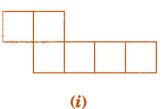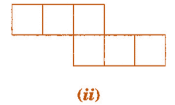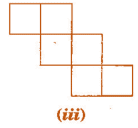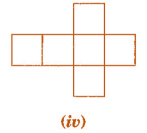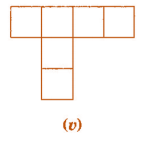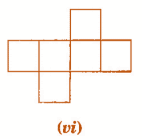Cube’s nets are (ii), (iii), (iv) and (vi).

Question 2:

Dice are cubes with dots on each face. Opposite faces of a die always have a total of seven dots on them.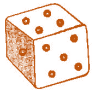Here are two nets to make dice (cubes); the numbers inserted in each square indicate the number of dots in that box.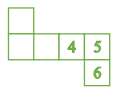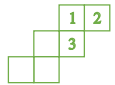Insert suitable numbers in the blanks, remembering that the number on the opposite faces should total to 7.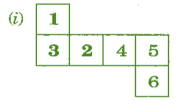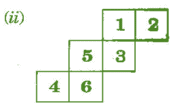Question 3: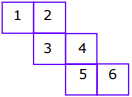No, this cannot be a net for a die.

Because one pair of opposite faces will have 1 and 4 on them and another pair of opposite faces will have 3 and 6 on them whose total is not equal to 7.

Question 4:

Here is an incomplete net for making a cube. Complete it in at least two different ways. Remember that a cube has six faces. How many faces are there in the net here? (Give two separate diagrams. If you like, you may use a squared sheet for easy manipulation.)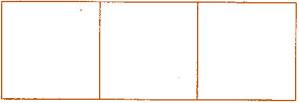There three faces are given: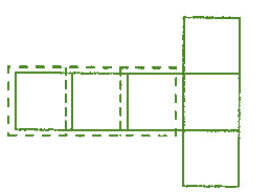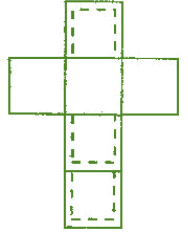Question 5:

Match the nets with appropriate solids: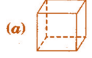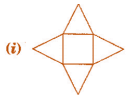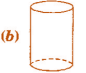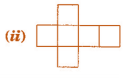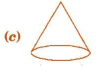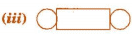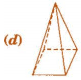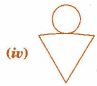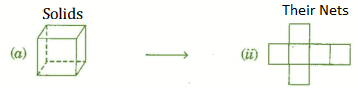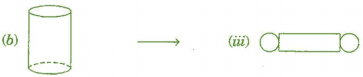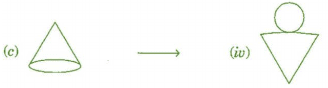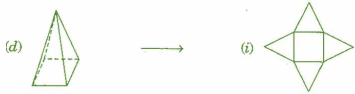Exercise 15.2

Question 1: Use isometric dot paper and make an isometric sketch for each one of the given shapes: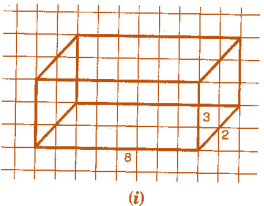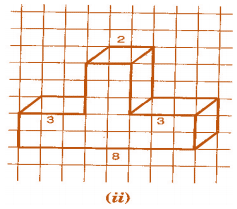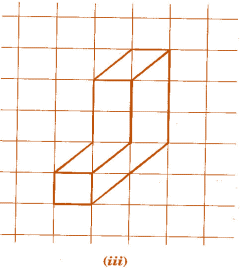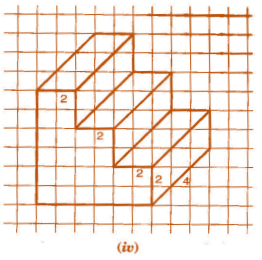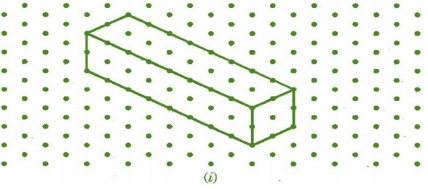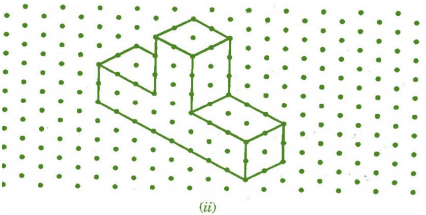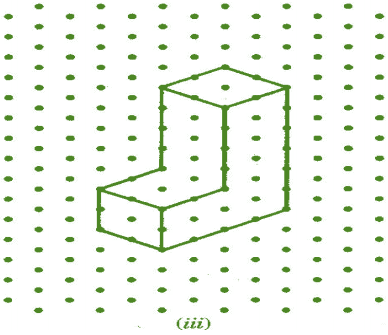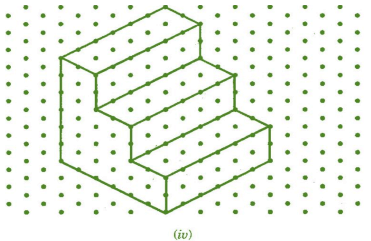Question 2:

The dimensions of a cuboid are 5 cm, 3 cm and 2 cm. Draw three different isometric sketches of this cuboid.

The dimensions of given cuboid are 5 cm, 3 cm and 2 cm: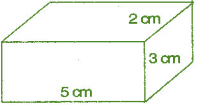Three different isometric sketches are: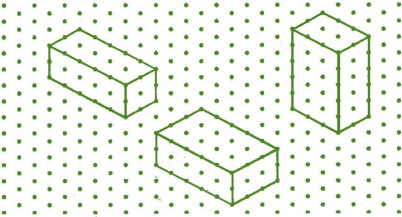Question 3:

Three cubes each with 2 cm edge are placed side by side to form a cuboid. Sketch an oblique or isometric sketch of this cuboid.

Oblique sketch: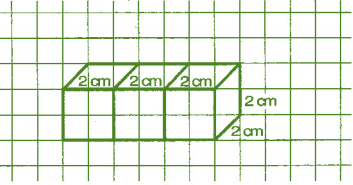Isometric sketch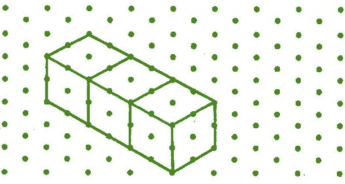Question 4:

Make an oblique sketch for each one of the given isometric shapes: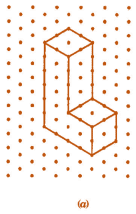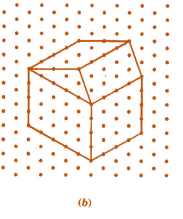Oblique sketches: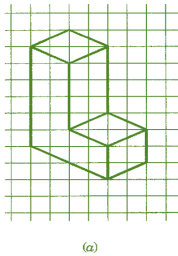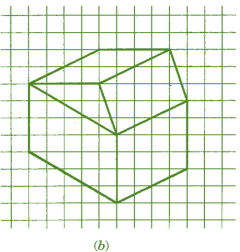Question 5:

Give

(i) an oblique sketch and (ii) an isometric sketch for each of the following:

(a) A cuboid of dimensions 5 cm, 3 cm and 2 cm. (Is your sketch unique?)

(b) A cube with an edge 4 cm long.

(a) A cuboid of dimensions 5 cm, 3 cm and 2 cm.

(i) Oblique sketch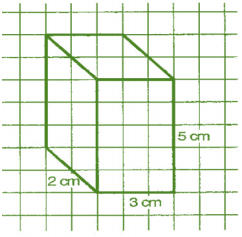(ii) Isometric sketch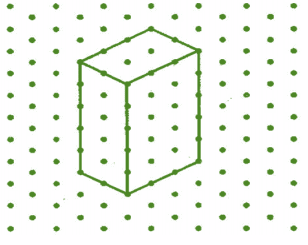(b) A cube with an edge 4 cm long.

(i) Oblique sketch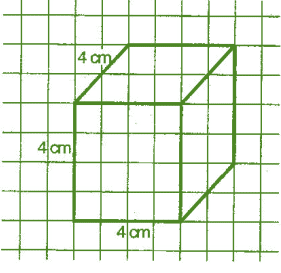(ii) Isometric sketch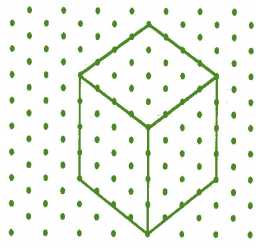Question 6:

An isometric sheet is attached at the end of the book. You could try to make on it some cubes or cuboids of dimensions specified by your friend.

Cubes and cuboids shapes on isometric sheet given below: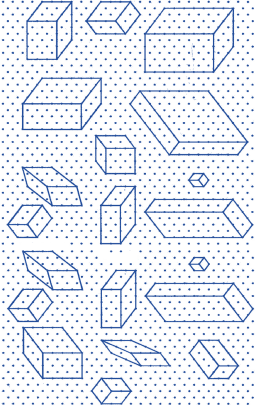You can also draw more shapes of cubes and cuboids.

Offer running on EduRev: Apply code STAYHOME200 to get INR 200 off on our premium plan EduRev Infinity!

,

,

,

,

,

,

,

,

,

,

,

,

,

,

,

,

,

,

,

,

,

;